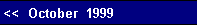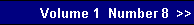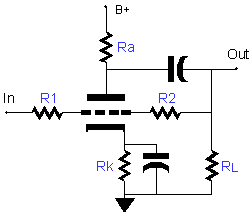desired, less than unity or more than unity gain. This is a great help when cascading stages and just a bit more gain is needed. Because the cathode is not floating at some high voltage, the issue of maintaining a low heater-to-cathode voltage difference does not arise.
 The first step is to determine the open-loop gain (a) of the Grounded Cathode amplifier and then the ratio of resistors R1 and R2. Thus,     R = Ra||RL||R2    a  =  muR/(muR + rp + (mu + 1)Rk),if Rk is bypassed, then     a  =  muR/(muR + rp)    Ratio = R2/R1and finally,    Gain =  aRatio/(a + Ratio + 1).Now that we have the gain, we can determine the output impedance.     Zo = (R||(rp + (mu +1)Rk))(Gain/a)if Rk is bypassed, then    Zo = (R||rp)(Gain/a).The Broskie Cathode Follower
 Plate Follower circuit
 The negatives for this buffer are that its bandwidth is compromised by the low pass filter created by resistor R1 working into the Miller Effect capacitance of the triode and the this same resistor defines the input impedance of the circuit and serves to load down the previous stage. Increasing the value of R1 unburdens the previous stage, but worsens the high frequency response. Additionally, the circuit requires three extra resistors and, potentially, a bypass capacitor for resistor Rk as well.The Math    Since the Plate Follower is functionally equivalent to the inverting Op-Amp circuit, the mistake that is commonly made is to assume that the gain is given by R2/R1. This formula works for Op-Amps as they have near infinite open loop gain, whereas the Grounded Cathode amplifier, which is at the core of this circuit, has a very finite gain that can never exceed the mu of the triode. Consequently, the formula for the gain of the Plate Follower must include the relatively weak gain of the triode used.
 Although this circuit was covered in the June Issue in the article on converting a balanced signal into single-ended one, it deserves to be reexamined as a buffer circuit.     As a balanced to SE converter, the circuit function is the subtract signal B for signal A. Because balanced signals consist of two phases, the function effectively becomes A + B. A signal common to both A and B, let us call it C, is canceled, as the function C - C obtains. Noise is usually equally shared between two balanced signals and is thus eliminated in the single-ended output. So far, the circuit mimics a transformer in function, which was the goal.       But this circuit, which I have been testing and I have found to work so very well that I have named it the "Broskie Cathode Follower," differs from a transformer in that it does not reflect impedances, but rather provides a low output impedance and a symmetrical current swing, i.e. it can  aggressively pull up or down like a White Cathode Follower.     The Broskie Cathode Follower is like a Cathode Follower wedded to a Plate Follower, but not quite.pg. 9